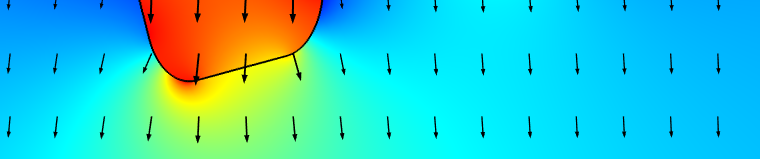## Electrostatics, Theory

電磁気学   静電気学

### Electrostatics in Free Space

Electrostatics is the subfield of electromagnetics describing an electric field caused by static (nonmoving) charges. Starting with free space, assuming a space charge density,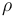, the relationship with the electric field,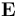, is:

(1)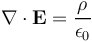where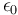is a universal constant of nature called the permittivity of free space.

This relationship means that, in electrostatics, the space charge density acts like a volume source. The charge-field relationship is not enough, but Maxwell's equations imply the additional requirement that the electric field is irrotational (curl free):

(2)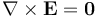which is the static version of Faraday's law.The static electric field,, surrounding two capacitor plates is an example of an irrotational vector field.

The static electric field,, surrounding two capacitor plates is an example of an irrotational vector field.

For an irrotational field, there exists a scalar potential, which leads to the definition of the electric potential,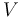:

(3)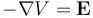The vector identity:

(4)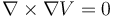is true for any sufficiently smooth scalar field forand ensures that the field is irrotational. The negative sign for the electric potential is an old convention.The electric potential field,, surrounding two capacitor plates.

The electric potential field,, surrounding two capacitor plates.

By combining the above, the information contained in Maxwell's equations for electrostatics can be encoded in just one equation:

(5)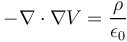The usefulness of this equation in engineering applications is limited due to its inability to represent dielectric materials. To remedy this problem, the theory is extended with the effects of induced polarization.

### Electrostatics in Dielectric Materials

An idealized dielectric material is characterized by the fact that it does not have any free charges but instead has bound charges. At the microscopic level, these bound charges can be displaced by an external electric field and result in induced electric dipoles. These induced electric dipoles are pairs of positive and negative charges that in some way align with the electric field. This results in an electric field inside a dielectric material that is different from that of free space. To get a macroscopic description of this phenomenon, it is convenient to introduce a polarization vector field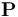, and a polarization charge density,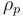. They are related by:

(6)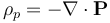The polarization effects locally modify the electric field on the inside of a material according to:

(7)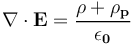or equivalently:

(8)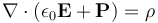Based on this, a new fundamental quantity can be introduced. The electric displacement field,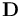, is defined as:

(9)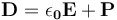Using this definition, the electrostatics equation, also known as Gauss's law, becomes:

(10)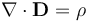To fully describe electrostatics phenomena, the condition that the electric field is irrotational (Faraday's law) still needs to hold. Since this condition is encoded in the electric potential, the equations of electrostatics can be combined into a single equation:

(11)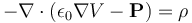The field around an object of higher permittivity than its surroundings. The images show a dielectric object,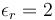, surrounded by air,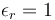, between two capacitor plates (not shown). The top and bottom electrodes have a positive and negative electric potential, respectively. The image to the left shows the magnitude of the electric field,, in color and its direction with arrows. The image to the right shows the magnitude of the electric displacement field,, in color and its direction with arrows. Red and blue represent a high and low magnitude value, respectively.

The field around an object of lower permittivity than its surroundings. The images show an air cavity,, surrounded by a dielectric material,, between two capacitor plates. The top and bottom electrodes have a positive and negative electric potential, respectively. The image to the left shows the magnitude of the electric field,, in color and its direction with arrows. The image to the right shows the magnitude of the electric displacement field,, in color and its direction with arrows. Red and blue represent a high and low magnitude value, respectively.

#### Linear Dielectric Materials

In electrostatics, one can frequently assume that the material is linear. This means that the polarization vector field is proportional to the electric field:

(12)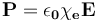where the constant of proportionality,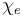, is the electric susceptibility.

In the case of an anisotropic material, the susceptibility can be a three-by-three tensor:

(13)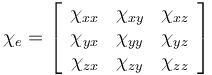Combine this with the relationship betweenand:

(14)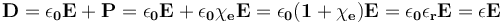and two new useful quantities can be introduced: the relative permittivity,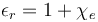, and the absolute permittivity,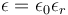.

Perhaps the most important constitutive relationship for linear dielectric materials is:

(15)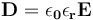An anisotropic susceptibility corresponds to an anisotropic relative permittivity:

(16)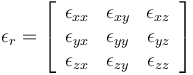This implies that for certain materials, theandfields may not be perfectly aligned.

Using the electrostatic potential, the fundamental equation for electrostatics in linear materials is:

(17)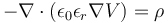### The Electrostatics Equations and Boundary Conditions at Material Interfaces

Gauss's law and Faraday's law can be seen as specifying conditions on the divergence and curl of the electric field, respectively. According to Helmholtz's theorem, this determines the electric field up to a constant. As a side note, this unknown constant is what ultimately makes it necessary to specify a ground level for the electric potential. At material interfaces, the divergence condition implies a condition on the normal component of the field and the curl condition implies a condition on the tangential component of the field. Material interfaces represent discontinuities, and to more easily understand what conditions to impose on boundaries, the corresponding integral forms are usually used. The boundary formulations are then derived by taking the limit of a shrinking closed surface (Gauss's law) and the limit of a shrinking closed contour (Faraday's law), respectively, which enclose a portion of the material interface.

This is summarized in the following table:

Equation Name Differential Form Integral Form Boundary Condition
Gauss's law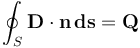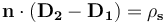Faraday's law (electrostatics),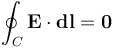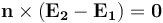,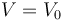where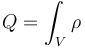is the volume charge and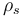is the surface charge at the material interface.

The simulation to the left shows the induced surface charge density on perfectly conducting metallic objects, while the simulation to the right shows the electric potentials. In the right image, red and blue represent a positive and negative potential, respectively. The top and bottom plates are held at fixed, but different, electric potentials. The middle closed surface has an induced constant floating potential, which, due to symmetry, is the average of the potentials of the plates. The surface charge density is distributed so that each metallic surface is an equipotential surface, an implication of Gauss's law.

It can be useful to summarize the meaning of these equations in words:

Equation Name Differential Form Integral Form Boundary Condition
Gauss's law All field lines start and end on charges. The total flux through a closed surface equals its enclosed charge. The surface charge at a material interface equals the jump in the normal component of the displacement field.
Faraday's law (electrostatics) The electric field is irrotational. The electric field is conservative. Across a material interface, the tangential component of the electric field is continuous.

It should be noted that for the time-varying case, the electric field is not irrotational, and Faraday's law gets an additional term corresponding to electromagnetic induction.Two charges of opposite signs are enclosed within the green and blue spheres. The electric field is visualized by cyan flux lines. The red sphere has no enclosed charge. Gauss's law states that the flux of the electric displacement field through the green sphere equals the enclosed charge, and similarly for the blue sphere. The red sphere with no enclosed charge has as many incoming field lines as outgoing ones, corresponding to a net zero flux.

Two charges of opposite signs are enclosed within the green and blue spheres. The electric field is visualized by cyan flux lines. The red sphere has no enclosed charge. Gauss's law states that the flux of the electric displacement field through the green sphere equals the enclosed charge, and similarly for the blue sphere. The red sphere with no enclosed charge has as many incoming field lines as outgoing ones, corresponding to a net zero flux.

### Electrostatic Energy

The electrostatic energy contained in a field can be expressed in many different ways. For a dielectric medium, the electrostatic energy in a volume,, expressed in field quantities is: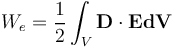where the electrostatic energy density is defined as: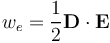Notice that the localization of electrostatic energy density has a limited physical justification (see also: The Feynman Lectures on Physics, Volume 2.

Another expression of electrostatic energy in terms of the volume charge densityand the local electric potential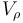is: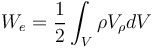and the two expressions for energy can be shown to be equivalent.

The concept of electrostatic energy is useful when computing electrostatic forces and capacitance values.

Published: February 14, 2019